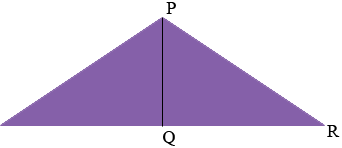SEARCH HOMEMath Central Quandaries & QueriesQuestion from Jo: Find the angle between the two sides of length 5 in an isosceles triangle that has one side of length 9 and two sides of length 5.Hi Jo,

I can help get you started.

Draw a line from the vertex $P$ of the triangle where the two sides of length 5 inches meet to the midpoint $Q$ of the opposite side.What do you know about triangle $PQR?$

PennyMath Central is supported by the University of Regina and The Pacific Institute for the Mathematical Sciences.